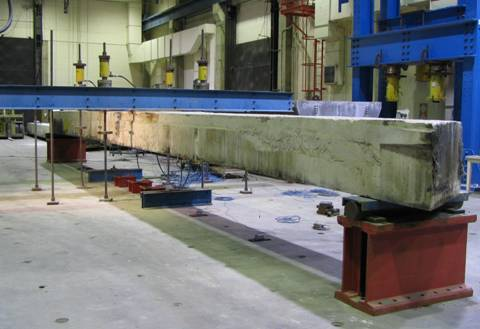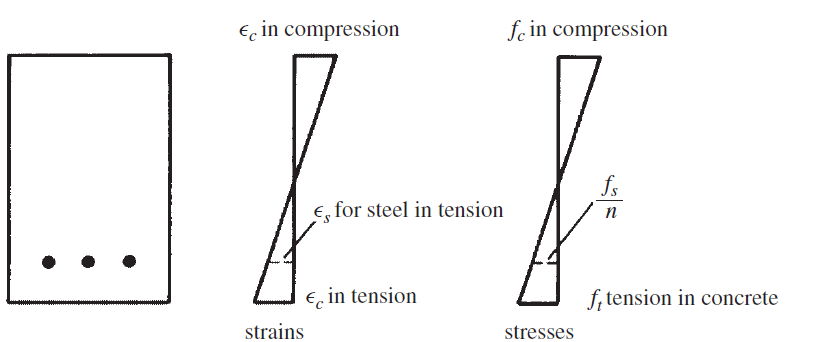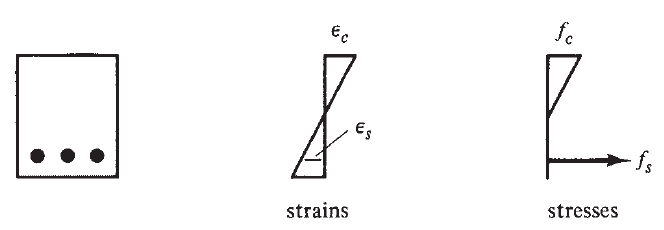## RC Beam Behavior Under LoadConcrete beam

Assuming that a small transverse load is placed on a concrete beam with tensile reinforcing and that the load is then gradually increased in magnitude until the beam fails. Through that process, the beam undergoes three distinct stages up to failure as indicated next:

1- Uncracked Concrete Stage

At small load range where the modulus of rupture (the bending tensile stress at which the concrete begins to crack) is higher than the tensile stresses on the beam, the beam cross section resists the bending with compression on one side and tension on the other.Uncracked concrete stage

2- Concrete Cracked–Elastic Stresses Stage

By increasing the load such that the modulus of rupture of the concrete is exceeded, cracks begin to develop in the bottom of the beam. The moment at which these cracks begin to form is referred to as the cracking moment, Mcr. As the load is further increased, these cracks quickly spread up to the vicinity of the neutral axis, and then the neutral axis begins to move upward. The cracks occur at those places along the beam where the actual moment is greater than the cracking moment.Now that the bottom has cracked, another stage is present because the concrete in the cracked zone obviously cannot resist tensile stresses—the steel must do it. This stage will continue as long as the compression stress in the top fibers is less than about one-half of the concrete’s compression strength, fc, and as long as the steel stress is less than its yield stress. The stresses and strains for this range are shown in next figure. In this stage, the compressive stresses vary linearly with the distance from the neutral axis or as a straight line. The straight-line stress–strain variation normally occurs in reinforced concrete beams under normal service-load conditions because at those loads, the stresses are generally less than 0.5 fc,.3- Beam Failure—Ultimate-Strength Stage

As the load is increased further so that the compressive stresses are greater than fc,, the tensile cracks move farther upward, as does the neutral axis, and the concrete compression stresses begin to change appreciably from a straight line. Assuming that the reinforcing bars have yielded, the stress variation is much like that shown in figureStage 3